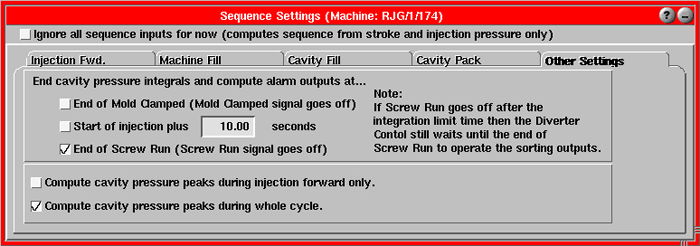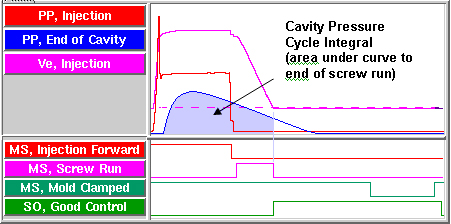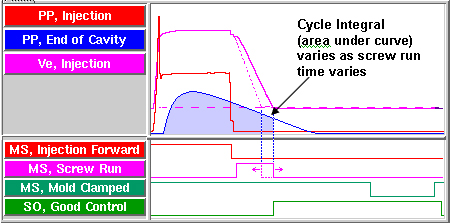Select Page

What is an Integral?

“Integral” is a term that comes from calculus making it naturally rather scary. Don’t worry. It roughly translates to “area”; as in simple geometry, area = width x length. In our business the areas in which we are most interested are the areas under the pressure (and volume) curves. These areas are the Cycle Integral values that the eDART computes. They are some of the most useful values in sorting parts, especially for dimensions.

Since these curves are not shaped like rectangles think of them as thousands of thin, vertical rectangles with the area under the curve being the sum of the areas of all of them. Like the area of a rectangle we need an overall “width”; that is to say: when should the integral start and when should it end? In the eDART the start is always the start of the cycle. The end of the Cycle Integral is what we call the “Integration Limit”. At that time the eDART does the math and supplies the sorting output to the part diverter (robot, conveyor, flipper chute etc).

How do you choose the integration limit on the eDART?

With release 8.4 of the eDART software the eDART sets the integration limit to the end of mold clamped. If you need to change that ending you can open the “Sequence Settings” and select the “Other Settings” tab.• As shown above the integration limit for Cycle Integral is set to the end of mold clamped (0 seconds before end).

• You can also choose to set the Cycle Integral limit to a fixed time period, regardless of sequence input signals.

• If both boxes are ticked then the Cycle Integral will end at the first of the two times.

• If neither box is ticked then the Cycle Integral ends at the end of Screw Run (as shown below).How should you choose the integration limit?

& Some automation equipment must have the signal computed before the mold opens; for example a flipper chute onto which the parts drop. then you can un-tick both boxes and let the integrals be computed at the end of screw run. This works only if there is no cavity pressure left when the screw stops (screw run goes off).

If there is pressure in the mold at the end of screw run then variations in the screw run time (which are quite common) will cause variations in the area (integral) even if the cavity curves are exactly the same from shot to shot.These variations are not really related to the part since the curves are the same.

Of course, if there was no pressure at the end then the height of the tiny “rectangles” in that region would be zero and not add to the integral at all.

If there is pressure at the end of screw run (as shown above) and your automation equipment requires an early signal then you can tick the first box and use a time before mold clamped. This assumes that the mold clamped time set on the machine is a fixed process time.

But… if the above is true and some machines have a mold clamped signal and some do not then set the integration limit to a fixed time (second tick box). This should be done after the process has been created, centered, is stable and validated to make good parts. If you do not do this then the integral will end at the end of Screw Run on those machines where Mold Clamped is missing.